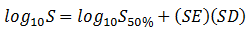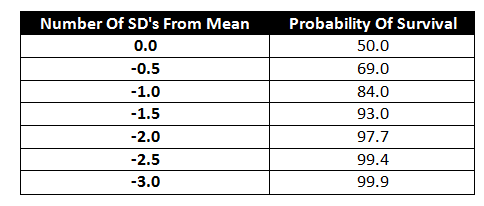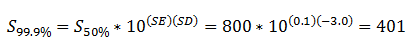# How to Account for Fatigue Data ScatterMay 15, 2015 By: Michael Bak

High cycle fatigue analysis of an engineering structure is performed by evaluating the stress range due to cyclic stresses that occur from given load conditions, and then using fatigue data of that material to determine cycles to failure.  Fatigue data is usually obtained by cycling test specimens at constant amplitude stresses until failure occurs.  These fatigue tests are repeated multiple times at different stress levels until an S-N curve that correlates stress range or amplitude (S) to number of cycles to failure (N) can be plotted.  An example is shown above.

Fatigue data contains significant scatter.  A test specimen cycled at a given amplitude will provide a range of failure cycles.  The standard technique of accounting for this scatter is to use a statistical approach and describe the scatter using probability density functions. A representation of this statistical distribution is shown in the figure below, taken from NASA Contractor Report 3697, Statistical Summaries of Fatigue Data for Design Purposes by P. H. Wirsching. Note from the figure that the probability density functions can describe the scatter in cycles at a given stress level (horizontal scatter), or scatter in stress at a given number of cycles (vertical scatter).

Probability Density Functions Used To Describe Scatter In S-N Fatigue Data

## fatigue scatter2.pngThe normal or Gaussian distribution is commonly used to define the probability distributions of the fatigue data.  Statistical analysis can be performed on the data to provide the mean and the standard deviation.

Once fatigue data is generated, and statistical analysis is performed on it, the fatigue data is typically provided using the mean curve, which represents a 50% probability of survival or average life, and the value of the standard deviation.  Since S-N data is plotted on logarithmic scales, the standard deviation value is usually called the "Standard Error of log10 N" or the "Standard Error of log10 S", and is often given the label SE.

The main reason to review and understand how fatigue scatter is characterized is that if the mean curve is used in life prediction, the cycles to failure predicted will represent 50% probability of survival. There are very few engineering applications where a one out of two chance of survival is acceptable!

Note that some S-N data is provided with a single curve, and the curve has already been shifted to account for a particular survival probability.  But it is clearly important to understand exactly what your fatigue data represents. For example, in nCode DesignLife, most fatigue properties in the built-in material library are defined with a 50% probability of survival curve and a standard error.  nCode provides an additional user input for the certainty of survival that will be used in the fatigue life calculation, but the default is 50%.

The equation used to shift the data, given below for the case of shifting the stress (S) when the standard error (SE) is provided, is:where SD is the number of standard errors used to shift the data.  The table below relates probability of survival to number of standard errors from mean.Scatter in fatigue data is often significant, as can be illustrated with a simple example.  Consider a fatigue specimen that is cycled with a stress range of 800 MPa, which will produce an average life of 100,000 cycles.  If the S-N data has a standard error (SE) of 0.1, the stress range that will provide a 99.9% probability of survival is 401 MPa, which is half of the average value:Thus, it is vitally important to know how the scatter in the fatigue data you use in your life predictions is defined, what probability of survival was assumed for the given S-N curve, and what probability of survival is acceptable for your application.  If you have any questions or comments, please let me know.